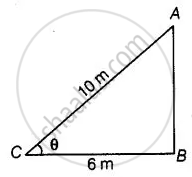# Foot of a 10 m long ladder leaning against a vertical wall is 6 m away from the base of the wall. Find the height of the point on the wall where the top of the ladder reaches. - Mathematics

Sum

Foot of a 10 m long ladder leaning against a vertical wall is 6 m away from the base of the wall. Find the height of the point on the wall where the top of the ladder reaches.

#### Solution

Let AB be a vertical wall and AC = 10 m is a ladder.

The top of the ladder reaches to A and distance of ladder from the base of the wall BC is 6 m.In right-angled ΔABC,

AC^2 = AB^2 + BC^2   ......[By pythagoras theorem]

⇒ (10)^2 = AB^2 + (6)^2

⇒ 100 = AB^2 + 36

⇒ AB^2 = 100 - 36 = 64

∴ AB = sqrt(64) = 8 cm

Hence, the height of the point on the wall where the top of the ladder reaches is 8 cm.

Concept: Application of Pythagoras Theorem in Acute Angle and Obtuse Angle
Is there an error in this question or solution?

#### APPEARS IN

NCERT Mathematics Exemplar Class 10
Chapter 6 Triangles
Exercise 6.3 | Q 15 | Page 69

Share